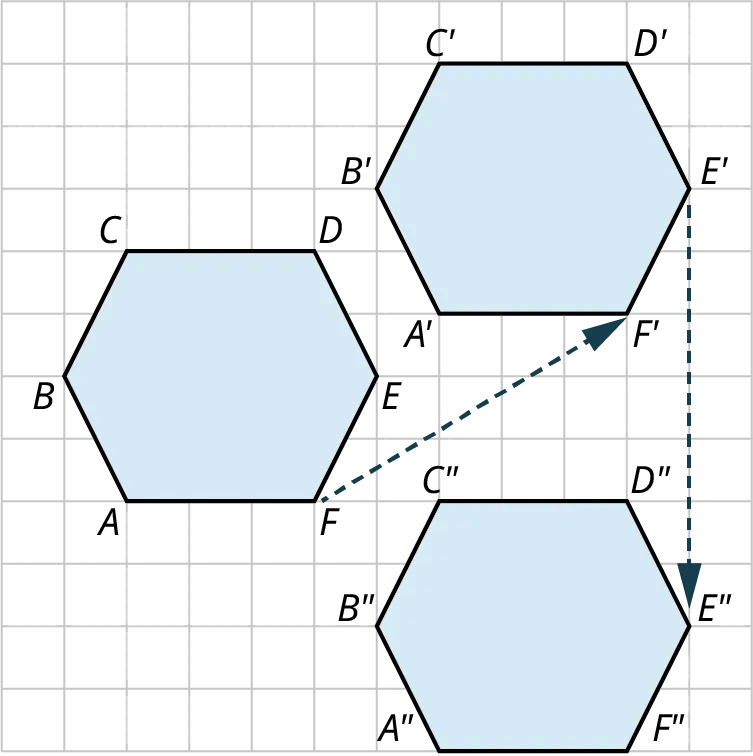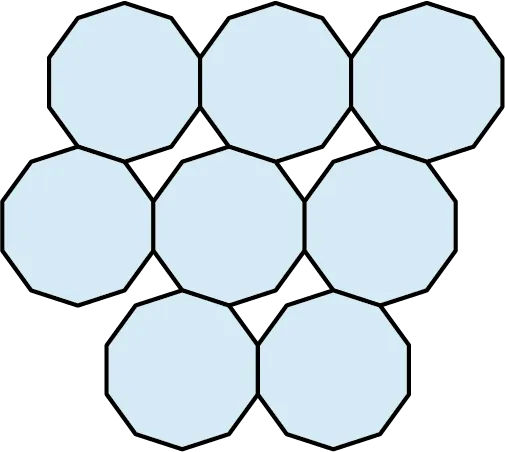Contemporary Mathematics

# Chapter 10

10.1
1.
$\overrightarrow {BD}$ is the ray that starts at point $B$ and extends infinitely in the direction of point $D$.
2.
$\overline {AB}$ represents the line segment that starts at point $A$ and ends at point $B$.
3.
$\overleftarrow {BA}$ represents the ray that starts at point $A$ and extends infinitely in the direction of point $B$.
4.
$\overleftrightarrow {AD}$ represents a line that contains the points $A$ and $D$. Notice the arrowheads on both ends of the line above $\mathit{AD}$, which means that the line continues infinitely in both directions.
10.2
1.
Answers will vary. One way ${\overline {AE}} ,\,{\overline {EI}} ,\,{\overline {IE}} ,\,{\overline {EB}} ,\,{\overline {BC}} ,\,{\overline {CD}} ,\,{\overline {DG}} \,$; Second way ${\overline {AH}} ,\,{\overline {HI}},\,{\overline {IE}} ,\,{\overline {EB}} ,\,{\overline {BC}} ,{\overline {CF}} ,\,{\overline {FG}}$.
10.3
1.
$\overleftrightarrow {RS}\parallel \overleftrightarrow {UV}$
$\overleftrightarrow {RS} \bot \overleftrightarrow {XY}$
$\overleftrightarrow {UV} \bot \overleftrightarrow {XY}$
10.4
1.
${\overline {AB}} \cap {\overline {BC}} = B$
2.
$\overrightarrow {BC} \cup \overleftarrow {CA} = \overleftrightarrow {AC}$
3.
${\overline {BC}} \cap {\overline {AC}} = {\overline {BC}}$
10.5
1.
Point $A$ is located at $( - 5, - 2)$; Point $B$ is located at $(- 3,4)$; Point $C$ is located at $(3, - 6)$; Point $D$ is located at $(5, - 2)$; Point $E$ is located at $(3,2)$; Point $F$ is located at $(5,5)$.
2.
Points $A$ and $B$ are on the straight line $\overleftrightarrow {AB}$.
3.
The line that begins at point $E$ in the direction of point $F$ is a ray, $\overrightarrow {EF}$.
4.
The line from point $C$ to point $D$ is a line segment $\overline {CD}$.
5.
Yes, this represents a plane. One reason is that the figure contains four points that are not on the line $\overleftrightarrow {AB}$.
10.6
1.
Plane $MNOP$ intersects with plane $OPQR$, and plane $OPQR$ intersects with plane $QRST$.
10.7
1.
Acute Angles Obtuse Angles Right Angles Straight Angles
$\angle AOB$
$\angle AOC$
$\angle BOC$
$\angle BOD$
$\angle COD$
$\angle DOE$
$\angle FOE$
$\angle AOE$
$\angle BOF$
$\angle COF$
$\angle AOD$
$\angle BOE$
$\angle DOF$
$\angle AOF$
10.8
1.
$\begin{array}{rcl}{5x}&{ = }&{{125^ \circ }} \\ {2x + 5} & { = } & {{55^ \circ }}\end{array}$
10.9
1.
$m\measuredangle (6x) = {24^ \circ}$, $m\measuredangle (9x) = {36^ \circ}$, $m\measuredangle (7x + 2) = {30^ \circ}$
10.10
1.
$m\measuredangle 4 = {67^ \circ }$, $m\measuredangle 1 = {113^ \circ }$, $m\measuredangle 3 = {113^ \circ }$
10.11
1.
${130^ \circ }$
10.12
1.
$m\measuredangle 5 = {120^ \circ}$, $m\measuredangle 4 = {120^ \circ}$, $m\measuredangle 8 = {120^ \circ}$
10.13
1.
$m\measuredangle 1 = {132^ \circ}$, $m\measuredangle 5 = {132^ \circ}$
10.14
1.
$m\measuredangle 5 = {56^ \circ }$
$m\measuredangle 6 = {62^ \circ }$
$m\measuredangle 7 = {118^ \circ }$
$m\measuredangle 8 = {62^ \circ }$
$m\measuredangle 9 = {118^ \circ }$
10.15
1.
$m\measuredangle x = {66^ \circ }$, $m\measuredangle (x + 1) = {67^ \circ }$, $m\measuredangle (x - 19) = {47^ \circ }$
10.16
1.
$m\measuredangle 1 = {35^ \circ }$; $m\measuredangle 2 = 2 = {85^ \circ }$; $m\measuredangle 3 = {60^ \circ }$
10.17
1.
Triangle $ABC$ is congruent to triangle $DEF$.
10.18
1.
SAS
10.19
1.
ASA
10.20
1.
The triangles are similar.
10.21
1.
$x = 6$, $y = 24$
10.22
1.
The tree is 86 feet high.
10.23
1.
60 ft
10.24
1.
rectangle
2.
pentagon
3.
heptagon
4.
parallelogram
10.25
1.
Shapes 1, 2, 4, and 6 are triangles; shape 3 is a pentagon; shape 5 is a parallelogram; and shape 7 is a rectangle.
10.26
1.
120 in
10.27
1.
22.4 in
10.28
1.
$a = {108^ \circ }$
The sum of the interior angles is ${540^ \circ }$.
10.29
1.
We have the sum of interior angles is ${360^ \circ }$. Then, $x = - 9.$ The other angles measure ${54^ \circ }$, ${111^ \circ }$, ${44^ \circ }$.
10.30
1.
$7(51.43) = {360^ \circ }$
10.31
1.
$C = 2(2.25)\pi = 14.14\text{ cm}$
10.32
1.
$r = 2.5\,\text{cm}$
10.33
1.
You need to buy 15.74 feet of trim.
10.34
1.
The translated hexagon has labels $A''$, $B''$, $C''$, $D''$, $E''$, $F''$.10.35
1.
Rotate the triangle about the rotation point ${90^ \circ }$ to the right three times.
10.36
1.
This tessellation could be produced with a reflection of the triangle vertically, then each triangle is rotated ${180^ \circ }$ and translated to the right.
10.37
1.
From the first square on the left, rotate the square ${30^ \circ }$ to the right, or $A1 \to A2$. Then, reflect the square over the horizontal, or $A2 \to A3$. Next, reflect all three squares over the vertical line. The lavender triangles comprise another pattern that tessellates, fits in with the squares, and fills the gaps.
10.38
1.
No
10.39
1.
Not by themselves, but by adding an equilateral triangle, the two regular polygons do tessellate the plane without gaps.
10.40
1.
We made a tessellation with a regular decagon (10 sides) and an irregular hexagon. We see that the regular decagon will not fill the plane by itself. The gap is filled, however, with an irregular hexagon. These two shapes together will fill the plane.10.41
1.
$8\,\text{cm}^2$
10.42
1.
108 ft2
10.43
1.
45 boxes at a cost of $2,025.00 10.44 1. $270\,{\text{i}}{{\text{n}}^2}$ 10.45 1. 13,671 square feet; cost is$19,872.95.
10.46
1.
$132\,{{\text{in}}^2}$
10.47
1.
${d_2} =10$
10.48
1.
40 in
10.49
1.
$A = 96.25\,{\text{c}}{{\text{m}}^2}$
10.50
1.
$30\,{\text{y}}{{\text{d}}^2}$
10.51
1.
$28.3\,{\text{c}}{{\text{m}}^2}$
10.52
1.
the 15-inch pizza
10.53
1.
$A = 21.875 + 4.8 = 26.7\,{\text{f}}{{\text{t}}^2}$
10.54
1.
$21.46\,{\text{c}}{{\text{m}}^2}$
10.55
1.
$300 10.56 1. $\begin{array}{rcl}{SA}&{ = }&{432\text{ cm}{^2}}\\{V}&{ = }&{540\text{ cm}{^3}}\\ \end{array}$ 10.57 1. $100\,\text{cm}{^2}$ 10.58 1. $\begin{array}{rcl} {SA}&{ = }&{336\text{ cm}{^2}}\\ {V}&{ = }&{480\text{ cm}{^3}}\\ \end{array}$ 10.59 1. $1{,}192\text{ ft}{^2}$ 10.60 1. $\begin{array}{rcl} {SA}&{ = }&{527.78\text{ cm}{^2}}\\ {V}&{ = }&{769.69\text{ cm}{^3}}\\ \end{array}$ 10.61 1. approximately 8 1/3 cans of soup 10.62 1. 6.25 ft by 6.25 ft 10.63 1. $1.26\,{\text{ft}}\,{\text{wide}}\, \times \,1.26\,{\text{ft}}\,{\text{long}}\, \times \,1.26\,{\text{ft}}\,{\text{high}},$$95.26
10.64
1.
$a = 5$
10.65
1.
1,140 ft
10.66
1.
The slanted distance will be 120.4 inches.
10.67
1.
The side lengths are $15,15\sqrt 3,30.$
10.68
1.
The ladder reaches 12 feet up the wall and sits $12\sqrt {3\,}{\text{ feet}}$ from the wall.
10.69
1.
Each side $x$ equals $x = 4\sqrt 2 = 5.66.$
10.70
1.
$\begin{array}{*{20}{l}} {r = 6.53} \\ {y = 4.2} \end{array}$
10.71
1.
$\alpha = {46.3^ \circ },\,\beta = {43.7^ \circ }$, $x = 5.73$
10.72
1.
2,241 ft
10.73
1.
$c = 8.06$, one angle is ${60^ \circ }$, and the other angle is ${30^ \circ }$.
10.74
1.
${11.9^ \circ }$
10.75
1.
46 ft

1.
The line containing point $D$ and point $A$ is a line segment from point $D$ to point $A$, $\overline{DA}$, or from point $A$ to point $D$, $\overline {AD}$.
2.
The line containing points $C$ and $B$ is a straight line that extends infinitely in both directions and contains points $C$ and $B$.
3.
This is a ray that begins at point $E$, although it does not contain point $E$, and extends in the direction of point $F$.
4.
${\overline {AB}} \cup {\overline {BD}} = \overline {AD}$. The union of line segment $\overline {AB}$ and the line segment ${\overline {BD}}$ contains all points in each line segment combined.
5.
$\overrightarrow {BD} \cap {\overline {BC}} = {\overline {BC}}$. The intersection of the ray $\overrightarrow {BD}$ and the line segment ${\overline {BC}}$ contains only the points common to each set, ${\overline {BC}}$.
6.
$\overrightarrow {BA} \cup \overrightarrow {BD} = \overleftrightarrow {AD}$. The union of the ray starting at point $B$ and extending infinitely in the direction of $A$ and the ray starting at point $B$ and extending infinitely in the direction of $D$ is the straight line extending infinitely in both directions containing points $A$, $B$, $C$, and $D$.
7.
Two lines are parallel if the distance between the lines is constant implying that the lines cannot intersect.
8.
Perpendicular lines intersect forming a ${90^ \circ }$ angle between them.
9.
Yes, because it contains a line and a point not on the line.
10.
straight
11.
obtuse
12.
right
13.
acute
14.
$m\measuredangle 1 = {149^ \circ }$ by supplementary angles with $\measuredangle {31^ \circ }.$
15.
$m\measuredangle 3 = {31^ \circ }$ by vertical angles with the angle measuring ${31^ \circ }.$
16.
$m\measuredangle 5 = {149^ \circ }$ by corresponding angles with $\measuredangle 1.$
17.
$x = {89^ \circ }$
18.
$x = {67^ \circ }$
19.
$x = {77^ \circ }$ and $y = {26^ \circ }$
20.
These are similar triangles, so we can solve using proportions.
 $\begin{array}{*{20}{rcl}}{\frac{3}{2}}&{ = }&{\frac{{(3 + 4)}}{x}} \\ {3x}&{ = }&{14} \\ {x}&{ = }&{\frac{{14}}{3}} \\ \end{array}$ $\begin{array}{*{20}{rcl}}{\frac{4}{2}}&{ = }&{\frac{{4 + y}}{{\frac{{14}}{3}}}} \\{\frac{{14}}{3}(4)}&{ = }&{2(4 + y)} \\{\frac{{56}}{3}}&{ = }&{8 + 2y} \\{56}&{ = }&{3(8 + 2y)} \\{56}&{ = }&{24 + 6y} \\{32}&{ = }&{6y} \\{\frac{{16}}{3}}&{ = }&{y}\end{array}$
Then, $x = \frac{{14}}{3}$ and $y = \frac{{16}}{3}$.
21.
Set up the proportions.
$\begin{array}{*{20}{rcl}}{\frac{6}{a}}&{ = }&{\frac{{12}}{{14}}} \\{6(14)}&{ = }&{12a} \\{84}&{ = }&{12a} \\{7}&{ = }&{a}\end{array}$
Thus, $t = 20$ and $a = 7.$
22.
pentagon
23.
octagon
24.
heptagon
25.
$6(5) = 30\text{ cm}$
26.
$S = \left( {6 - 2} \right){180^ \circ } = {720^ \circ }$
27.
${120^ \circ }$
28.
$\begin{array}{*{20}{rcl}}{360}&{=}&{152 + 9x + (5x + 1) + (x + 12)}\\{}&{=}&{15x + 165}\\{195}&{=}&{15x}\\{13}&{=}&{x}\\{x + 12}&{=}&{25^ \circ },\,9x = {117^ \circ },\,5x + 1 = {66^ \circ }\end{array}$
29.
$C = 2\pi (3) = 6\pi = 18.85\text{ cm}$
30.
The patterns are repeated shapes that can be transformed in such a way as to fill the plane with no gaps or overlaps.
31.
Starting with the triangle with the point labeled $A$, the triangle is translated point by point 3 units to the right and 3 units up to point $A'$. Then, the triangle labeled $A'$ is translated 3 units to the right and 3 units up to point $A''$.
32.
The triangle is rotated about the rotation point $180^\circ$ to vertex $B'$.
33.
The dark triangle is reflected about the vertical line showing the light back, and then reflected about the horizontal line. The pattern is repeated leaving a white diamond between the shapes.
34.
3.3.3.3.3.3
35.
7.5 cm2
36.
25 ft
37.
$168\,{\text{c}}{\text{m}^2}$
38.
$64.5\,{\text{c}}{{\text{m}}^2}$
39.
201.1 in2
40.
$116.38\,{\text{i}}{{\text{n}}^2}$
41.
$706.86\,{\text{i}}{{\text{n}}^2}$
42.
$386.6\,\text{cm}{^2}$
43.
$416\,\text{in}{^2}$
44.
$640\,\text{in}{^3}$
45.
$942.48\,\text{cm}{^2}$
46.
$1,570\,\text{cm}{^3}$
47.
$511.35\,\text{in}{^2}$
48.
$\begin{array}{*{20}{l}} {a = 2.5} \\ {b = 2.5\sqrt 3 } \end{array}$
49.
$\begin{array}{*{20}{l}} {b = 10} \\ {c = 10\sqrt 2 } \end{array}$
50.
$b = 6$
51.
24.2 ft
52.
$c = 11.7{\text{ cm}}$
53.
$x = 14.3{\text{ m}}$
Order a print copy

As an Amazon Associate we earn from qualifying purchases.